# The Performance Gains of Using MADlib for In-Database Analytics

### July 4, 2014 | Alan

If you are analysing a big data set you will likely hit the limits of in-memory analytics. Carrying out the same analysis in-database, can be an effective and time saving solution. This is where MADlib comes in.

We will look at training a linear regression model with the airlines data set, and then using this to predict the airtime of a flight dependent on multiple known variables. In Statistics, linear regression is a method for modelling a relationship between a scalar dependent variable y and one or more independent explanatory variables x. When there is more than one explanatory variable this is known as multiple linear regression. We will also plot the resulting models using R.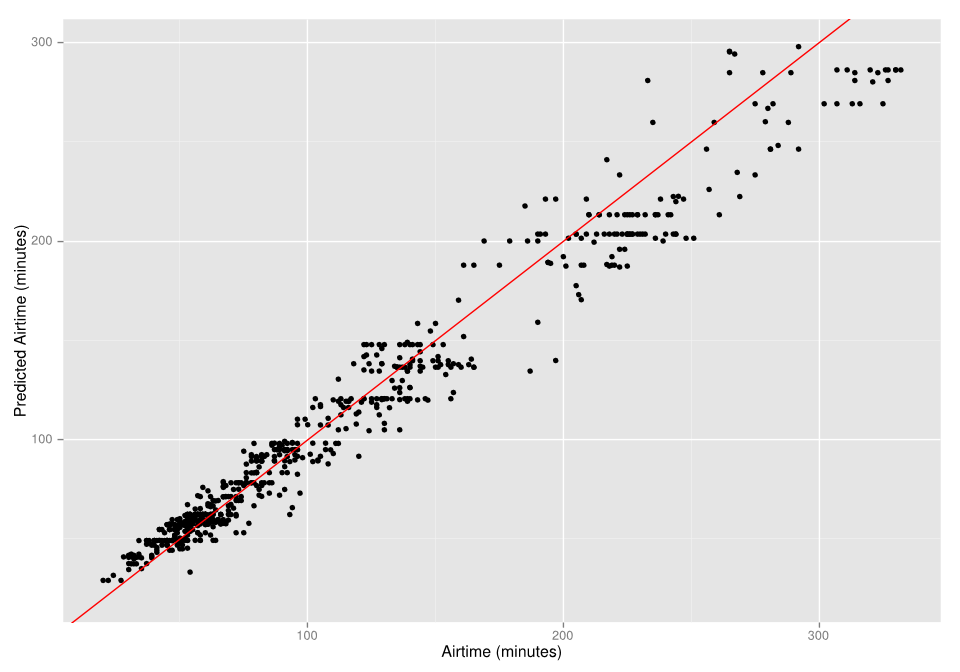### Data Source

The data set contains information about all flight arrival and departure details for all commercial flights within the USA from 1987 to 2008. There are almost 120 million rows in total. This is an open data set and can be found on the  ASA site.

### Learning Outcomes

After reading this blog you will have a better understanding of how you can utilise the capabilities of MADlib for big data analytics.

### Step 1 – Fit a Linear Model to a Sample Data Set

Firstly we’ll work through the example supplied in the MADlib user documentation. Open up a sql file in the AnalytiXagility platform. To do this go to the workfiles tab and click on the + button.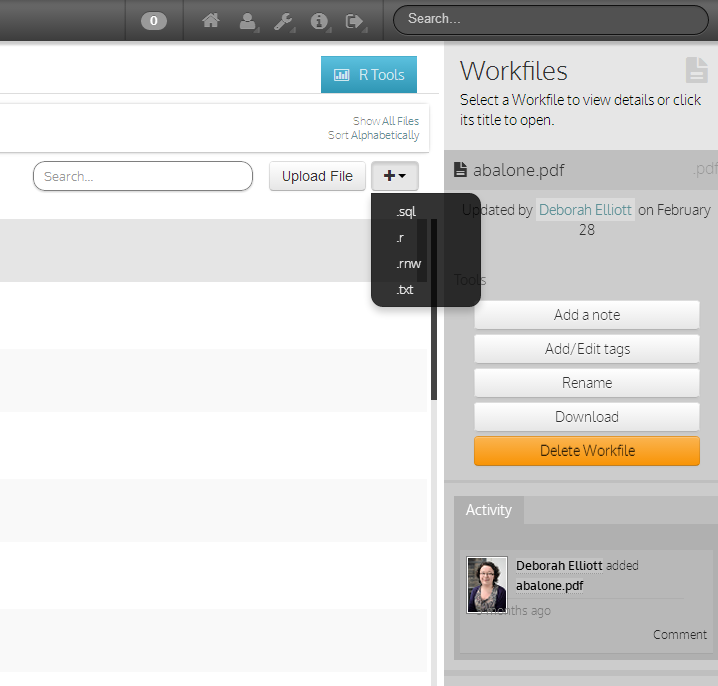Let’s create a sample data set to use for this first example.

CREATE TABLE houses (id INT, tax INT, bedroom INT, bath FLOAT, price INT,
size INT, lot INT);
INSERT INTO houses VALUES
( 1,  590, 2,   1,  50000,  770, 22100),
( 2, 1050, 3,   2,  85000, 1410, 12000),
( 3,   20, 3,   1,  22500, 1060,  3500),
( 4,  870, 2,   2,  90000, 1300, 17500),
( 5, 1320, 3,   2, 133000, 1500, 30000),
( 6, 1350, 2,   1,  90500,  820, 25700),
( 7, 2790, 3, 2.5, 260000, 2130, 25000),
( 8,  680, 2,   1, 142500, 1170, 22000),
( 9, 1840, 3,   2, 160000, 1500, 19000),
(10, 3680, 4,   2, 240000, 2790, 20000),
(11, 1660, 3,   1,  87000, 1030, 17500),
(12, 1620, 3,   2, 118600, 1250, 20000),
(13, 3100, 3,   2, 140000, 1760, 38000),
(14, 2070, 2,   3, 148000, 1550, 14000),
(15,  650, 3, 1.5,  65000, 1450, 12000);

To execute a sql statement you can either execute the whole file or selected text. Highlight the code you wish to execute and click on the run selected button: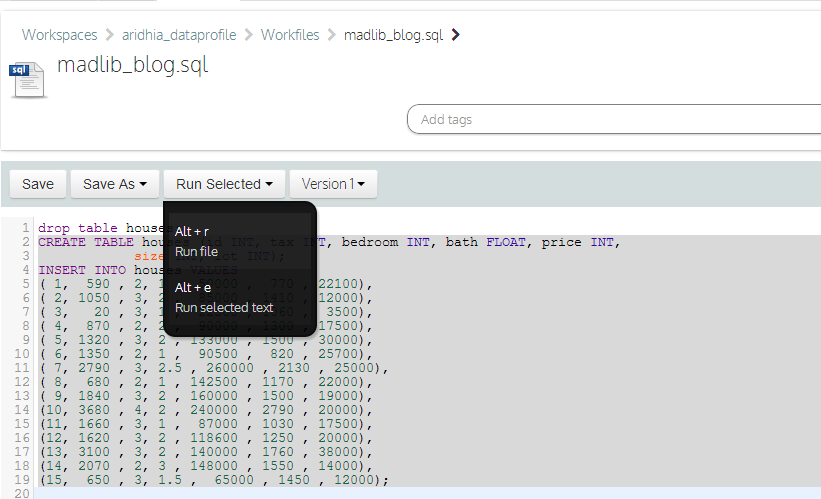Now lets train a regression model. We’ll start with a single regression for all the data and also record how long this takes to execute:

EXPLAIN ANALYSE
'houses_linregr',
'price',
'ARRAY[1, tax, bath, size]');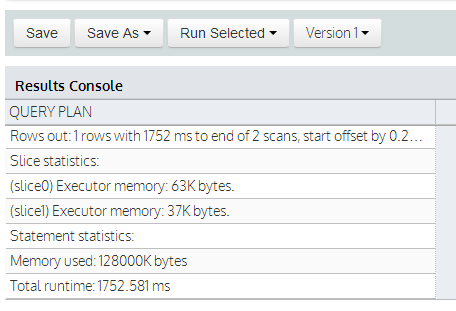Here we supply the source table, output table, dependent variable and independent variables. This takes 1-2 seconds to execute as expected. Let’s also generate three output models, one for each value of bedroom.

SELECT madlib.linregr_train( 'houses',
'houses_linregr_bedroom',
'price',
'ARRAY[1, tax, bath, size]',
'bedroom');

Now we can examine the resulting models by selecting the contents of the table:

SELECT * FROM houses_linregr;

However its much easier to read if we unnest the results:

SELECT unnest(ARRAY['intercept','tax','bath','size']) as attribute,
unnest(coef) as coefficient,
unnest(std_err) as standard_error,
unnest(t_stats) as t_stat,
unnest(p_values) as pvalue
FROM houses_linregr;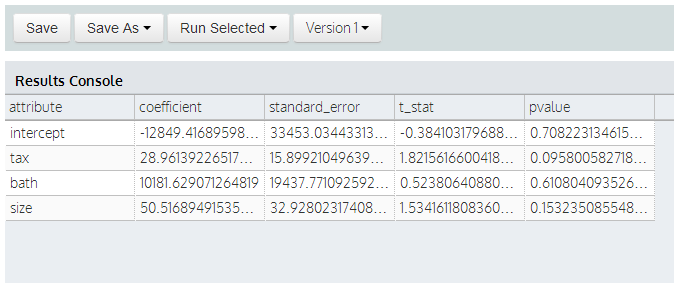Finally we can use the prediction function to evaluate the residuals:

SELECT houses.*,
m.coef
) as predict,
price -
m.coef
) as residual
FROM houses, houses_linregr m;


### Step 2 – Use a Much Bigger Data Set!

You may have been wondering in Step 1: “Why can’t I just do this in R?”. And for the size of data set above you easily could. However, we can now scale up to a data set with 120 million rows and MADlib will handle this without a grumble, whereas this would be a task next to impossible using in-memory analytics. The airlines data set will be used to demonstrate this. We’ll start with a simple model to predict the airtime of a flight using distance as the only explanatory variable and again record how long this takes to execute:

EXPLAIN ANALYSE
'airlines_linregr_onevar',
'airtime',
'ARRAY[1, distance]');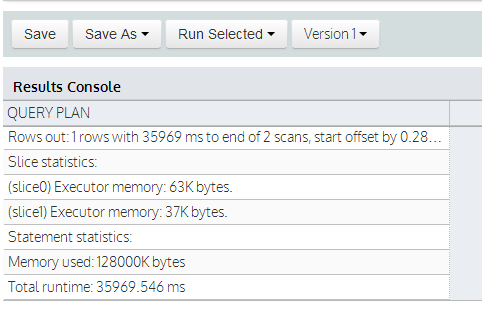This takes about 35 seconds – so roughly 35 times longer for a data set that is more than one million times bigger! And then look at the results using either of the following two queries:

SELECT * FROM airlines_linregr_onevar;

SELECT unnest(ARRAY['intercept','distance']) AS attribute,
unnest(coef) AS coefficient,
unnest(std_err) AS standard_error,
unnest(t_stats) AS t_stat,
unnest(p_values) AS pvalue
FROM airlines_linregr_onevar;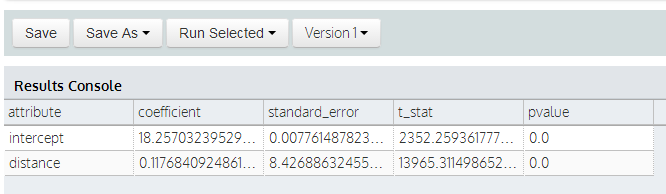The low standard errors for both coefficients and p-values suggest that airtime is dependent on both these variables. Using the prediction function we can calculate the predicted airtimes and compare these with the known airtimes:

SELECT airlines.*,
m.coef
) as predict,
airtime -
m.coef
) as residual
FROM airlines, airlines_linregr_onevar m;

Let’s plot a sample of the known values against the predicted airtime values in R to assess the fit. Firstly create a small sample of the data above and store in the database:

SELECT airlines.*,
m.coef
) as predict,
airtime -
m.coef
) as residual
into airlines_predict_onevar
FROM airlines, airlines_linregr_onevar m
limit 1000;

Open up the R console in the AnalytiXagility platform by clicking on the R tools tab on the right hand side, and read in the sample data set:

airlines_sample_onevar <- xap.read_table("airlines_predict_onevar")

To generate a plot we need to load the ggplot2 library. We then create a scatterplot of the known airtime vs. the predicted airtime and overlay the line y=x. If the model predicts airtime perfectly then all the data points would lie on this line. For more information about creating plots using ggplot2 see our blog on the Fundamentals of ggplot2 explained.

library(ggplot2)

p <- qplot(airtime, predict, data = airlines_sample_onevar)
p + geom_abline(intercept = 0, slope = 1, colour="red") +
xlab("Airtime (minutes)") +
ylab("Predicted Airtime (minutes)")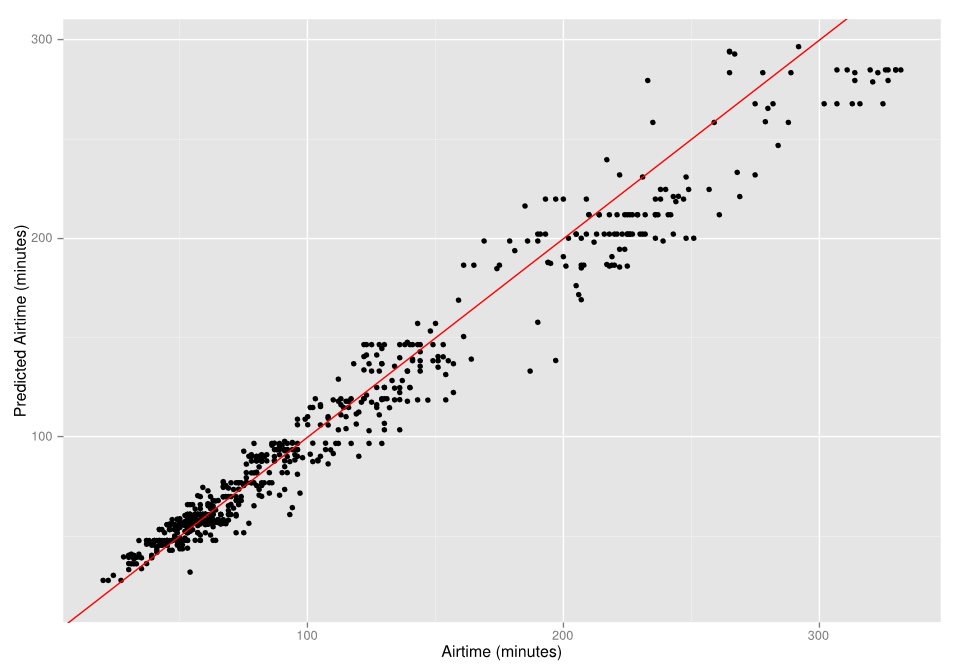From our plot we can see that our model appears to fit the data reasonably well. However we want to investigate whether any additional fields in the airlines data set would be significant explanatory variables. Lets go back into the database and repeat the above code, but with additional parameters:

EXPLAIN ANALYSE
'airlines',
'airlines_linregr',
'airtime',
'ARRAY[1, distance, dayofweek, month]');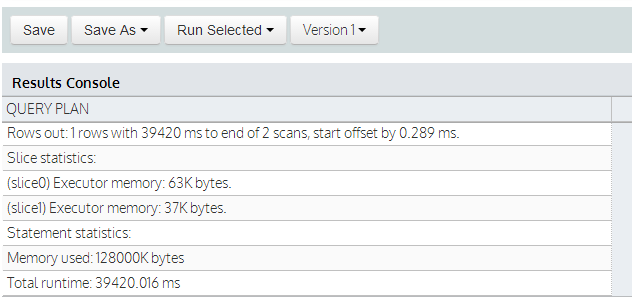Even with these additional parameters it only takes about 39 seconds to execute! Let’s look at the results:

SELECT * FROM airlines_linregr;

SELECT unnest(ARRAY['intercept','distance','dayofweek','month']) as attribute,
unnest(coef) as coefficient,
unnest(std_err) as standard_error,
unnest(t_stats) as t_stat,
unnest(p_values) as pvalue
FROM airlines_linregr;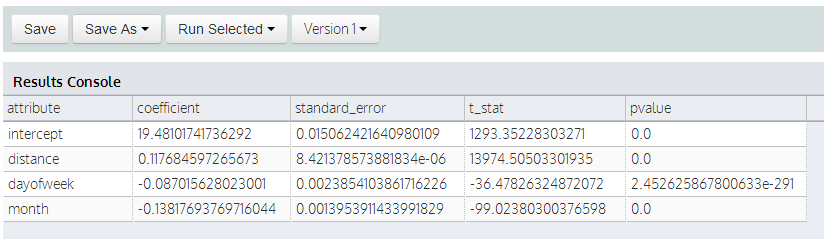Finally we can use the prediction function to evaluate the residuals:

SELECT airlines_madlib.*,
m.coef
) as predict,
airtime -
m.coef
) as residual
FROM airlines, airlines_linregr m;

And then plot a sample of this data as before in the R console, by first creating a sample of 1000 rows and saving it to the database:

SELECT airlines.*,
m.coef
) as predict,
airtime -
m.coef
) as residual
into airlines_predict_multiplevar
FROM airlines, airlines_linregr m
limit 1000;

And then repeat the code above in the R console to produce the following plot:

library(ggplot2)

p <- qplot(airtime, predict, data = airlines_sample_multiplevar)
p + geom_abline(intercept = 0, slope = 1, colour="red") +
xlab("Airtime (minutes)") +
ylab("Predicted Airtime (minutes)")